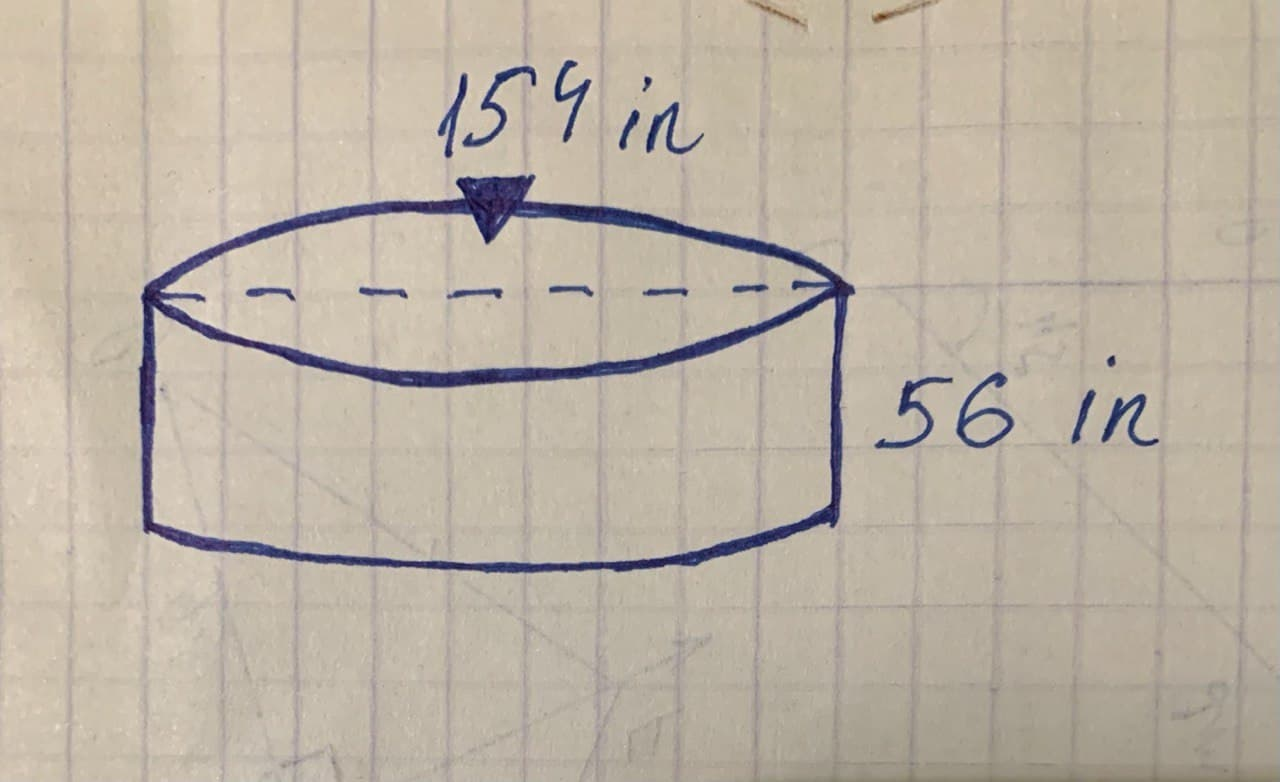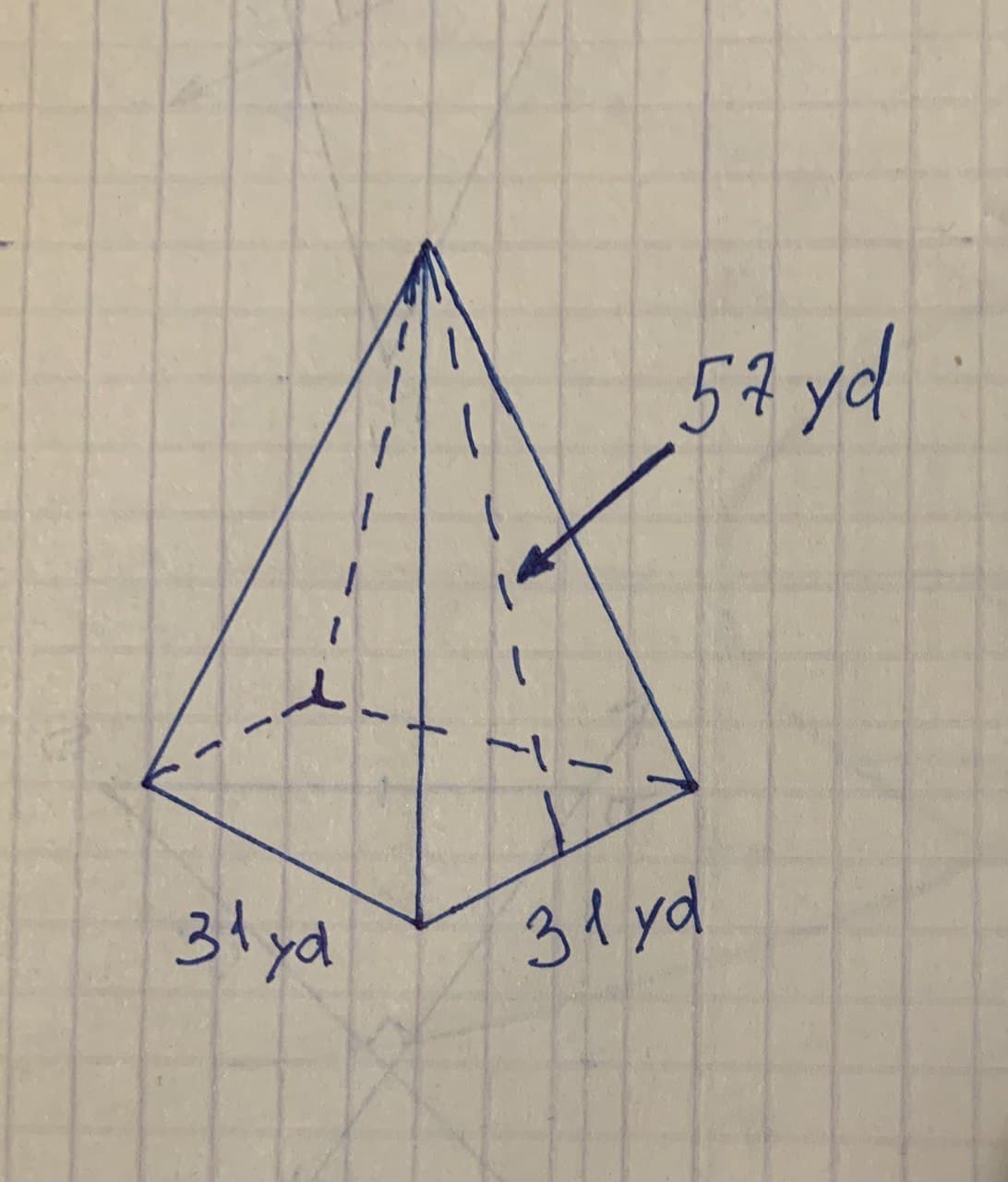# What is the surface area of this cylinder? FSP19610806971.jpgFSZ Use \pi\approx3.14 andTammy Fisher 2021-11-23 Answered
What is the surface area of this cylinder?Use $\pi \approx 3.14$ and round your answer to the nearest hundresth.
What is the surface area of this rectangular pyramid?You can still ask an expert for help

• Questions are typically answered in as fast as 30 minutes

Solve your problem for the price of one coffee

• Math expert for every subject
• Pay only if we can solve itElizabeth Witte

ans.
Total surface area of given cylinder $=2\pi \gamma h+2\pi {\gamma }^{2}$
Radius of given cylinder $=\frac{154}{2}=77$ in
By Putting values in formula $c\therefore$
$⇒2×3.14×77×56+2×3.14×77×77$
$⇒27079.36+37234.12$
$⇒64313.48i{n}^{2}⇒64313i{n}^{2}$

b=length of base
s=slent height
By Putting values in formula  $c\therefore$
$⇒2×31×57+31×31$
$⇒3534+961$
$⇒4495y{d}^{2}$